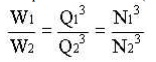Home | | Fluid Mechanics and Machinery | Centrifugal pump

# Centrifugal pump

If the mechanical energy is converted into pressure energy by means of centrifugal force acting on the fluid, the hydraulic machine is called centrifugal pump.

CENTRIFUGAL PUMP

Working principle

If the mechanical energy is converted into pressure energy by means of centrifugal force acting

on the fluid, the hydraulic machine is called centrifugal pump. The centrifugal pump acts as a reversed of an inward radial flow reaction turbine

Performance Characteristics of Pumps

The fluid quantities involved in all hydraulic machines are the flow rate (Q) and the head (H), whereas the mechanical quantities associated with the machine itself are the power (P), speed (N), size (D) and efficiency (h ). Although they are of equal importance, the emphasis placed on certain of these quantities is different for different pumps. The output of a pump running at a given speed is the flow rate delivered by it and the head developed. Thus, a plot of head and flow rate at a given speed forms the fundamental performance characteristic of a pump. In order to achieve this performance, a power input is required which involves efficiency of energy transfer. Thus, it is useful to plot also the power P and the efficiency h against Q.

Over all efficiency of a pump (h ) = Fluid power output / Power input to the shaft = rgHQ / P

Type number or Specific speed of pump, nS = NQ1/2 / (gH)3/4 (it is a dimensionless number)

Centrifugal pump Performance

In the volute of the centrifugal pump, the cross section of the liquid path is greater than in the impeller, and in an ideal frictionless pump the drop from the velocity V to the lower velocity is converted according to Bernoulli's equation, to an increased pressure. This is the source of the discharge pressure of a centrifugal pump.

If the speed of the impeller is increased from N1 to N2 rpm, the flow rate will increase from Q1 to Q2 as per the given formula:The head developed(H) will be proportional to the square of the quantity discharged, so thatThe power consumed(W) will be the product of H and Q, and, thereforeThese relationships, however, form only the roughest guide to the performance of centrifugal pumps.

Characteristic curves

Pump action and the performance of a pump are defined interms of their characteristic curves. These curves correlate the capacity of the pump in unit volume per unit time versus discharge or differential pressures. These curves usually supplied by pump manufacturers are for water only.

These curves usually shows the following relationships (for centrifugal pump).

·        A plot of capacity versus differential head. The differential head is the difference in pressure between the suction and discharge.

·        The pump efficiency as a percentage versus capacity.

·        The break horsepower of the pump versus capacity.

The net poisitive head required by the pump versus capacity. The required NPSH for the pump is a characteristic determined by the manufacturer.

Centrifugal pumps are usually rated on the basis of head and capacity at the point of maximum efficiency.

Study Material, Lecturing Notes, Assignment, Reference, Wiki description explanation, brief detail
Mechanical : Fluid Mechanics And Machinery : Pumps : Centrifugal pump |